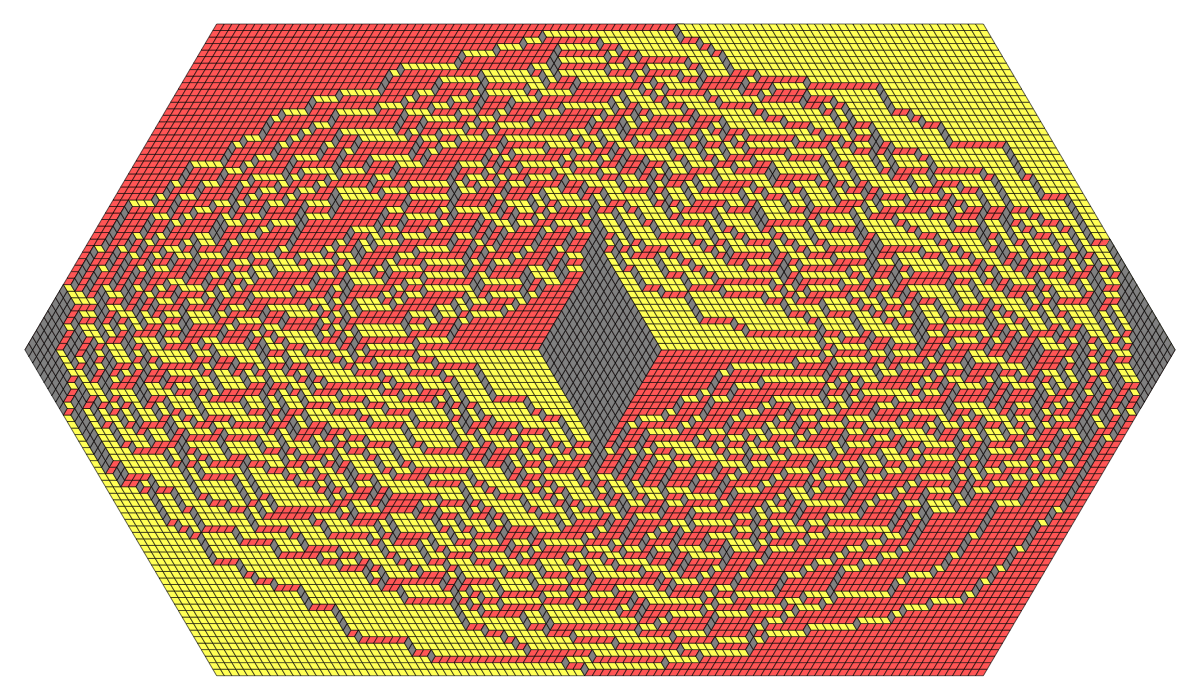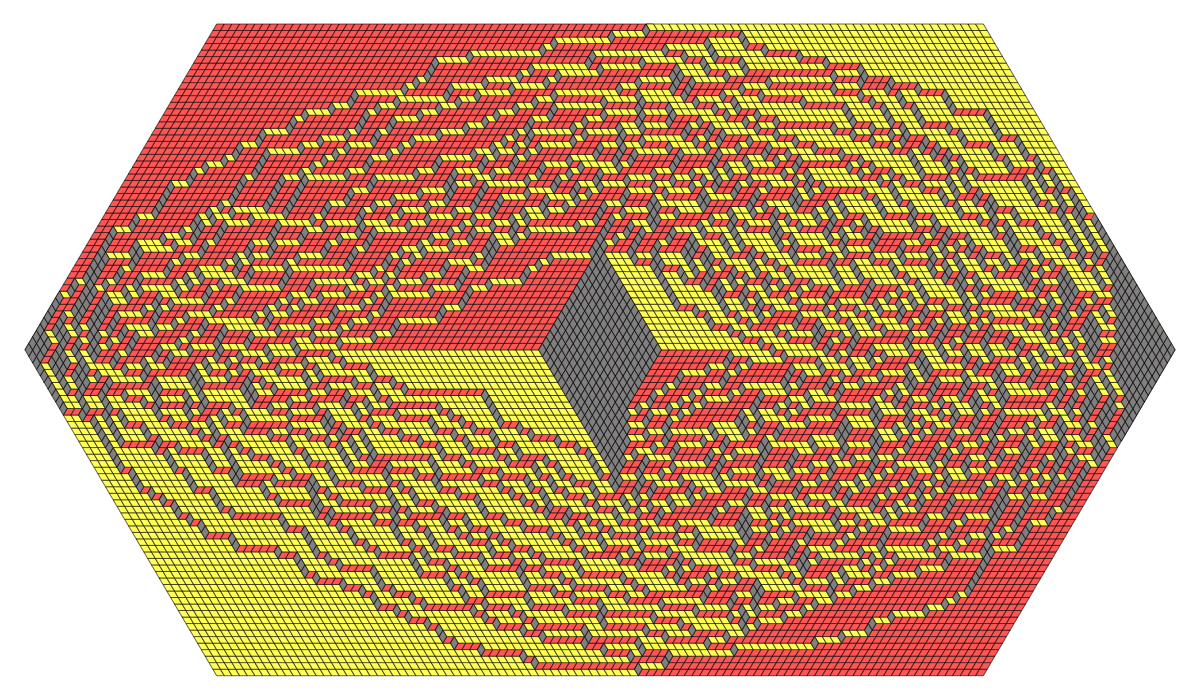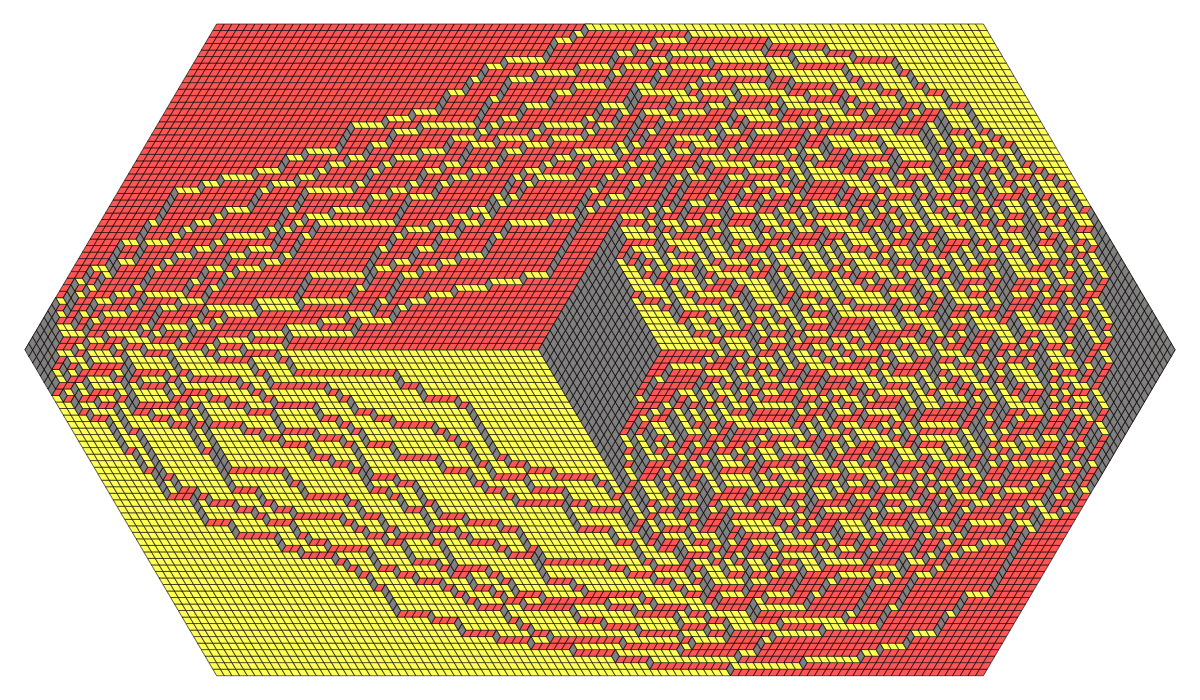# Holey hexagons with holes at different heights

#### Leonid Petrov

What happens if we sample a uniformly random tiling of a hexagon with a hole, but place the hole at different heights? (Thanks to MR for the request to sample these examples.)

### Data file format

The data file is a list of lists of lists in Mathematica-readable format, of the form $$\{ \lambda(1),\lambda(2),\ldots,\lambda(T) \},$$ where each $\lambda(t)$ is a list of weakly interlacing integer coordinates of the form $$\{ \{ 47,0,0,0,\ldots \},\{ 50,47,0,0,\ldots \} , \{ 50,49,47,0,\ldots \} ,\ldots, \} .$$ This list is a square array, and each $\lambda(i)$ is appended by zeroes.

## code • (Main GitHub repo)

(note: parameters in the code might differ from the ones in simulation results below)

## simulation results

1. ##### Tiling with a symmetric hole • (data: 57 KB) • (graphics: 8.7 MB)
Hexagon of size 100, hole size 15, shift 0.2. ##### Tiling with a skewed hole • (data: 57 KB) • (graphics: 8.7 MB)
Hexagon of size 100, hole size 15, shift 4.3. ##### Tiling with an even more skewed hole • (data: 57 KB) • (graphics: 8.7 MB)
Hexagon of size 100, hole size 15, shift 8.